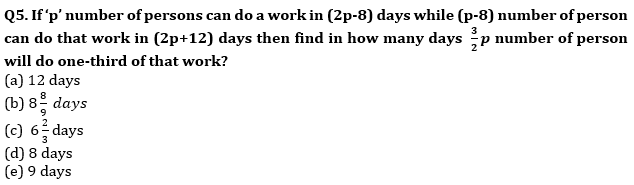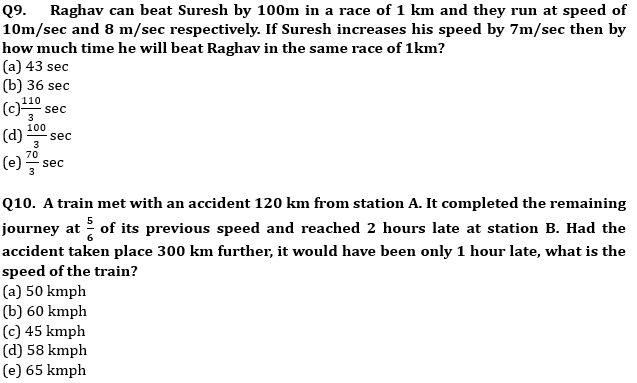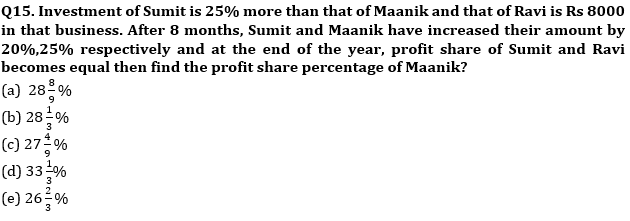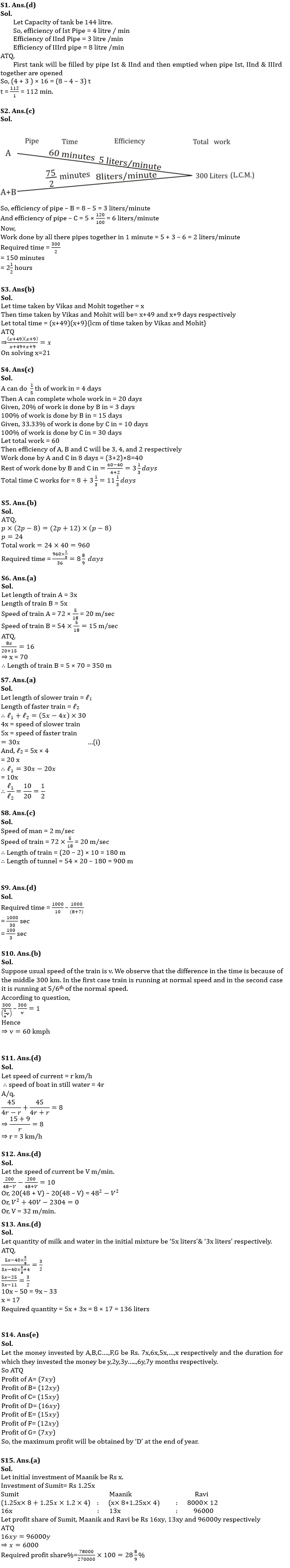Latest Banking jobs   »   Quantitative Aptitude Quiz For ECGC PO...

# Quantitative Aptitude Quiz For ECGC PO 2023 – 03rd May

Q1. A pipe can fill a tank in 36 minutes & another pipe can fill it in 48 minutes, but a third Pipe can empty it in 18 minutes. The first two pipes are kept open for 16 minutes in the beginning then the third Pipe is also opened. In what time is the cistern emptied?
(a) 120 min
(b) 80 min
(c) 96 min
(d) 112 min
(e) 144 min

Q2. There are three pipes-A, B & C. A & B are filling pipes and C is emptying pipe. Pipe-A alone can fill the tank in 1 hour & pipe-C is 20% more efficient than pipe-A. If pipe-A & B working together fill the tank in 75/2 minutes, then find in how much time pipe-A, B & C working together can fill the tank?
(a) 6 hours
(b) 4.5 hours
(c) 2.5 hours
(d) 3 hours
(e) 5 hours

Q3. Vikas can complete a work in 49 more days than in which Vikas and Mohit together can do the same work and Mohit can do the same work in 40 days less than that of Vikas. Find in how much time Mohit and Vikash can complete whole work together.
(a) 40 days
(b) 21 days
(c) 23 days
(d) can ’t Be détermined.
(e) 35 days.

Q4. A can do 1/5th of work in 4 days, B and C can do 20% and 33⅓% of work in 3 and 10 days respectively. If A and C work for 8 days and remaining work was done by B and C together, then find no of days C worked for.
(a) 3⅓ days
(b) 8⅓ days
(c) 11⅓ days
(d) 5⅓ days
(e) 6⅓ daysQ6. Ratio between length of trains A and B is 3 : 5. Speed of train A is 72 km/h and that of train B is 54 km/h & they are running opposite to each other. If train A crosses train B in 16 seconds then find length of train B.
(a) 350 m
(b) 250 m
(c) 450 m
(d) 150 m
(e) 320 m

Q7. The ratio of speed of two trains which are running in the same direction is 4 : 5. The train having higher speed crosses the second train in 30 seconds and a pole in 4 seconds respectively. Find the ratio of their lengths.
(a) 1 : 2
(b) 2 : 3
(c) 3 : 5
(d) 4 : 5
(e) 6 : 7

Q8. A train crosses a man, who is running in the same direction of train at the speed of 2m/sec. in 10 seconds. The same train crosses a tunnel in 54 seconds. If speed of train is 72 km/h then what is the length of tunnel?
(a) 850 m
(b) 800 m
(c) 900 m
(d) 750 m
(e) 650 mQ11. Speed of a boat in still water is 300% more than the speed of current. The boat takes a total time of 8 hours to cover a distance of 45 km upstream and 45 km in downstream both. Find speed of current.
(a) 2.5 km/h
(b) 2 km/h
(c) 4 km/h
(d) 3 km/h
(e) 5 km/h

Q12. A man can swim 48 m/min in still water. He swims 200 m against the current and 200 m with the current. If the difference between the time taken in both the cases is 10 min, then what is the speed of the current?
(a) 30 m/min
(b) 31 m/min
(c) 29 m/min
(d) 32 m/min
(e) 26 m/min

Q13. A mixture of milk and water is in ratio 5 : 3. If 40 liters of mixture is taken out and 4 liters of pure water is added to the mixture, then percentage of water in the mixture becomes 40%. Find the initial quantity of mixture.
(a) 148 liters
(b) 144 liters
(c) 150 liters
(d) 136 liters
(e) 140 liters

Q14. A,B,C…,F,G invested money in the ratio of 7:6:5:….2:1. The duration for which they invested the money is in the ratio of 1:2:3….6:7. Who will obtain maximum profit at the end of year?
(a) A
(b) G
(c) B
(d) F
(e) DSolutions## FAQs

### What is the total duration of ECGC PO for objective?

The duration of ECGC PO for objective is 140 minutes

#### Congratulations!Union Budget 2023-24: Free PDF